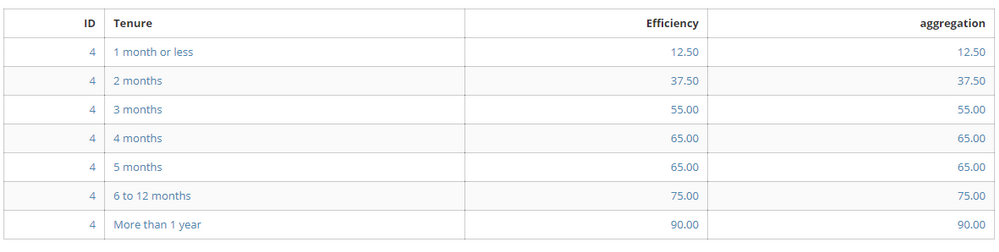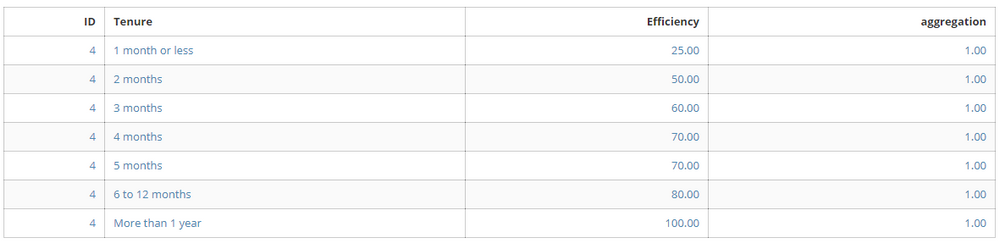Aggregating a column in Beast Mode

Is it possible to aggregate an entire column in a beast mode calculation within analyzer? (I have found videos explaining this within a SQL ETL, but not as a calculated field in analyzer).

For example: In the table below I want to divide the value in the "efficiency" column by the averge of all values in the column. Right now, the "aggregation" column is a calculated field with the formula =AVG('Efficiency'). It returns the value in the "Efficiency" column, so if I were to divide, I would always get "1." But I want each efficiency value divided by 57.14 - which is the average of all efficiency values.Is this possible with a Beast mode calculation, or do I need to edit the ETL? If I do need to edit the ETL, the card using this aggregation is the third frame of a drill path. Will the path be affected?

Thanks for the help!

Tagged:

• Hi @rgbuckley,

The calculation below should do the trick:

'Efficiency' / AVG('Efficiency')

Let me know how that goes

Cheers,

Eric

• Hi Eric,

Thanks for reacing out. When I do the calculation you suggest, the calculated field always returns 1, since it is taking the efficiency value divivded by the average of that one efficiency value. I need to aggregate the column before dividing. Thoughts?•